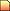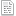# how to get the minimum value

Discussion in 'Programming General' started by PsychicFreak, Aug 22, 2010.

how to get the minimum value
1.#1 - Aug 22, 2010 at 12:27 AM
2. Joined:
Jun 6, 2008
Posts:
53
Referrals:
0
Sythe Gold:
0

### PsychicFreakMemberhow to get the minimum value

#include <stdio.h>
#include <conio.h>

int maximum (int, int, int, int, int);
int minimum (int, int, int, int, int);

int main()
{
int a, b, c, d, e;

printf("Enter five integers:");
scanf( "%d%d%d%d&d", &a, &b, &c, &d, &e );
printf( "Maximum is: %d\n", maximum( a, b, c, d, e ) );
printf( "minimum is: %d\n", minimum( a, b, c, d, e ) );

getch();
return 0;

}

int maximum( int v, int w, int x, int y, int z)
{
int max = v;
max = v;

if (w > max)
max = w;

if (x > max)
max = x;

if (y > max)
max = y;

if (z > max)
max = z;

return max;
}

int minimum( int v, int w, int x, int y, int z)
{
int min = v;
min = v;

if (w > min)
min = w;

if (x > min)
min = x;

if (y > min)
min = y;

if (z > min)
min = z;

return min;
}

how can iget the min value?? help

3.#2 - Aug 22, 2010 at 2:01 AM
4. Joined:
Apr 3, 2010
Posts:
364
Referrals:
0
Sythe Gold:
0how to get the minimum value

Make integer "min" = 20000 (or a high number)

Turn your inputted numbers into an array of say 10 integers.

Start a for loop for x < 10 starting from 0, adding 1.

Inside put: if (min > number[x]) min = number[x];

done.